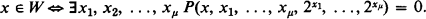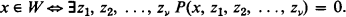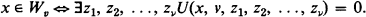Hostname: page-component-588bc86c8c-pkxkl Total loading time: 0 Render date: 2023-11-30T14:53:46.793Z Has data issue: false Feature Flags: { "corePageComponentGetUserInfoFromSharedSession": true, "coreDisableEcommerce": false, "useRatesEcommerce": true } hasContentIssue false

# Universal diophantine equation

Published online by Cambridge University Press:  12 March 2014

## Extract

In 1961 Martin Davis, Hilary Putnam and Julia Robinson  proved that every recursively enumerable set W is exponential diophantine, i.e. can be represented in the formHere P is a polynomial with integer coefficients and the variables range over positive integers.

In 1970 Ju. V. Matijasevič used this result to establish the unsolvability of Hilbert's tenth problem. Matijasevič proved  that the exponential relation y = 2x is diophantine This together with  implies that every recursively enumerable set is diophantine, i.e. every r.e. set Wcan be represented in the formFrom this it follows that there does not exist an algorithm to decide solvability of diophantine equations. The nonexistence of such an algorithm follows immediately from the existence of r.e. nonrecursive sets.

Now it is well known that the recursively enumerable sets W1, W2, W3, … can be enumerated in such a way that the binary relation xWv is also recursively enumerable. Thus Matijasevič's theorem implies the existence of a diophantine equation U such that for all x and v,Type
Research Article
Information
The Journal of Symbolic Logic , September 1982 , pp. 549 - 571

## Access options

Get access to the full version of this content by using one of the access options below. (Log in options will check for institutional or personal access. Content may require purchase if you do not have access.)

## References

#### REFERENCES

Baker, A., Contributions to the theory of diophantine equations. I, Philosophical Transactions of the Royal Society of London, Series A, vol. 263 (1968), pp. 173191.Google Scholar
Davis, Martin, Putnam, Hilary and Robinson, Julia, The decision problem for exponential diophantine equations, Annals of Mathematics, vol. 74 (1961), pp. 425436 = Matematika, vol. 8 (5) (1964), pp. 69–79.Google Scholar
Davis, Martin, Matijasevič, Yuri and Robinson, Julia, Hilbert's tenth problem. Diophantine equations : positive aspects of a negative solution, Proceedings of the Symposium on the Hilbert Problems (De Kalb, Illinois), May 1974, American Mathematical Society, Providence, R.I., 1976, pp. 323378.Google Scholar
Davis, Martin, Hilbert's tenth problem is unsolvable, American Mathematical Monthly, vol. 80 (1973), pp. 233269.Google Scholar
Jones, J.P., Undecidable diophantine equations, Bulletin of the American Mathematical Society (New Series), vol. 3, no. 2 (1980), pp. 859862.Google Scholar
Jones, J.P. and Matijasevič, Ju. V., A new representation for the symmetric binomial coefficient and its applications, Les Annales des Sciences Mathématiques du Québec (to appear).Google Scholar
Jones, J.P., Three universal representations of recursively enumerable sets, this Journal, vol. 43 (1978), pp. 335351. MR 58, 16226.Google Scholar
Jones, J.P., Diophantine representation of Mersenne and Fermat primes, Acta Arithmetica, vol. 35 (1978), pp. 209221.Google Scholar
Jones, J.P., Sato, D., Wada, H. and Wiens, D., Diophantine representation of the set of prime numbers, American Mathematical Monthly, vol. 83 (1976), pp. 449464. MR 54, 2615.Google Scholar
Kosovskiǐ, N.K., Ondiophantine representation of the sequence of solutions of Pell's equation, Zapiski Naučnyh Seminarov Leningradskogo Otdelenija Matematičeskogo Instituta im. V.A. Steklova Akademii Nauk SSSR, vol. 20 (1971), pp. 4959 (Russian); English transl., Journal of Soviet Mathematics, vol. 1 (1973), pp. 28–35.Google Scholar
Matijasevič, Ju. V., Enumerable sets are diophantine, Doklady Akademii Nauk SSSR, vol. 191 (1970), pp. 279282 (Russian); English transl., Soviet Mathematics Doklady, vol. 11 (1970), pp. 354–357.Google Scholar
Matijasevič, Ju. V., On recursive unsolvability of Hilbert's tenth problem, Proceedings of the IVth International Congress on Logic, Methodology and Philosophy of Science (Bucharest 1971), North-Holland, Amsterdam, 1973, pp. 89110. MR 57, 5711.Google Scholar
Matijasevič, Ju. V., Diophantine sets, Uspehi Matematičeskih Nauk, vol. 27 (1972), pp. 185222 (Russian); English transl, Russian Mathematical Surveys, vol. 27 (1972), 124–164.Google Scholar
Matijasevič, Ju. V., Arithmetical representation of enumerable sets with a small number of quantifiers, Zapiski Naučnyh Seminarov Leningradskogo Otdelenija Mathematiceskogo Instituto im. V.A. Steklova Akademii Nauk SSSR, vol. 32 (1972), pp. 7784 (Russian); English transl., Journal of Soviet Mathematics, vol. 6(1976), No. 4, pp. 410–416.Google Scholar
Matijasevič, Ju. V. and Robinson, Julia, Reduction of an arbitrary diophantine equation to one in 13 unknowns, Acta Arithmetica, vol. 27 (1975), pp. 521553.Google Scholar
Matijasevič, Ju. V., A new proof of the theorem on exponential diophantine representation of enumerable sets, Zapiski Naučnyh Seminarov Leningradskogo Otdelenija Mathematičeskogo Instituto im. V.A. Steklova Akademii Nauk SSSR, vol. 60 (1976), pp. 7589 (Russian); English translation, Journal of Soviet Mathematics, vol. 14 (1980), pp. 1475–1486.Google Scholar
Matijasevič, Ju. V., A class of primality criteria formulated in terms of the divisibility of binomial coefficients, Zapiski Naučhnyh Seminarov Leningradskogo Otdelenija Mathematičeskogo Instituta im. V.A. Steklova Akademii Nauk SSSR, vol. 67 (1977), pp. 167183 (Russian); English translation, Journal of Soviet Mathematics, vol. 16 (1981), pp. 874–884.Google Scholar
Matijasevič, Ju. V., Primes are non-negative values of polynomial in 10 variables, Zapiski Naučnyh Seminarov Leningradskogo Otdelenija Mathematičeskogo Instituto im. V.A. Steklova Akademii Nauk SSSR, vol. 68 (1977), pp. 6282 (Russian); English translation, Journal of Soviet Mathematics, vol. 15 (1981), pp. 33–44.Google Scholar
Matijasevič, Ju. V., Some purely mathematical results inspired by mathematical logic, Foundations of mathematics and computabiliiy theory (Butts, and Hintakka, , editors), D. Reidel Publishing Co., Dordrecht-Holland, 1977, pp. 121127.Google Scholar
Matijasevič, Ju. V., Algorithmic unsolvability of exponential diophantine equations in three unknowns, Studies in the theory of algorithms and mathematical logic, Nauka, Moscow, 1979, pp. 6978; English transl, available from Department of Mathematics and Statistics, University of Calgary.Google Scholar
Robinson, Julia, Hilbert's tenth problem, Proceedings of the Symposium on Pure Mathematics, vol. 20, American Mathematical Society, Providence, R.I., 1971, pp. 191194.Google Scholar
Siegel, Carl L., Zur theorie der quadratischen formen, Nachrichten der Akademie der Wissenschaften in Göttingen. II. Mathematisch-Physikalische Klasse (1972), No. 3, pp. 2146.Google Scholar
Kummer, E.E., Über die Ergänzungssätze zu der Allgemeiner Reziprozitätsgesetzen, Journal für die Reine und Angewandte Mathematik, vol. 44 (1852), pp. 93146.Google Scholar
Robinson, Julia, Diophantine decision problems, MAA Studies in Mathematics, vol. 6 (1969), [Studies in Number Theory (W.J. Leveque, editor)], pp. 76–116.Google Scholar
Robinson, Julia, Existential definability in arithmetic, Transactions of the American Mathematical Society, vol. 72 (1952), pp. 437449 = Matematika, vol. 8 (5) (1964), pp. 3–14. MR 14, 4.Google Scholar
Singmaster, D., Notes on binomial coefficients I, II, III, Journal of the London Mathematical Society, vol. 8 (3) (1974) pp. 545–548, 549–554, 555560.Google Scholar